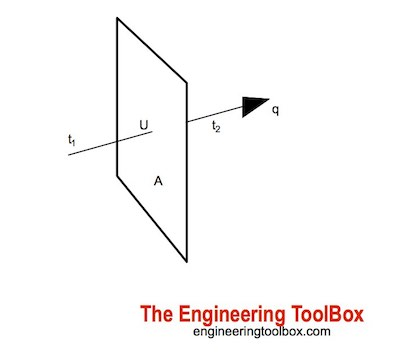Engineering ToolBox - Resources, Tools and Basic Information for Engineering and Design of Technical Applications!

# Conductive Heat Transfer

## Heat transfer takes place as conduction in a solid if there is a temperature gradient

Conduction as heat transfer takes place if there is a temperature gradient in a solid or stationary fluid medium.

With conduction energy transfers from more energetic to less energetic molecules when neighboring molecules collide. Heat flows in direction of decreasing temperatures since higher temperatures are associated with higher molecular energy.Conductive heat transfer can be expressed with "Fourier's Law"

q = (k / s) A dT

= U A dT                               (1)

where

q = heat transfer (W, J/s, Btu/hr)

k = Thermal Conductivity of material (W/m K or W/m oC, Btu/(hr oF ft2/ft))

s = material thickness (m, ft)

A = heat transfer area (m2, ft2)

U = k / s

=  Coefficient of Heat Transfer (W/(m2K), Btu/(ft2 h oF)

dT = t1 - t2

= temperature gradient - difference - over the material (oC, oF)

#### Example - Conductive Heat Transfer

A plane wall is constructed of solid iron with thermal conductivity 70 W/moC. Thickness of the wall is 50 mm and surface length and width is 1 m by 1 m. The temperature is 150 oC on one side of the surface and 80 oC on the other.

The conductive heat transfer through the wall can be calculated

q = [(70 W/m oC) / (0.05 m)] [(1 m) (1 m)] [(150 oC) - (80 oC)]

= 98000 (W)

= 98 (kW)

### Conductive Heat Transfer Calculator.

This calculator can be used to calculate conductive heat transfer through a wall. The calculator is generic and can be used for both metric and imperial units as long as the use of units is consistent.

k - thermal conductivity (W/(mK), Btu/(hr oF ft2/ft))

A - area (m2, ft2)

t1 - temperature 1 (oC, oF)

t2 - temperature 2 (oC, oF)

s - material thickness (m, ft)

### Conductive Heat Transfer through a Plane Surface or Wall with Layers in Series

The heat conducted through a wall with layers in thermal contact can be calculated as

q = dT A / ((s1 / k1) + (s2 / k2) + ... + (sn / kn))                                (2)

where

dT = t1 - t2

= temperature difference between inside and outside wall (oC, oF)

Note that heat resistance due to surface convection and radiation is not included in this equation. Convection and radiation in general have major impact on the overall heat transfer coefficients

#### Example - Conductive Heat Transfer through a Furnace Wall

A furnace wall of 1 m2 consist of 1.2 cm thick stainless steel inner layer covered with 5 cm outside insulation layer of insulation board. The inside surface temperature of the steel is 800 K and the outside surface temperature of the insulation board is 350 K. The thermal conductivity of the stainless steel is 19 W/(m K) and the thermal conductivity of the insulation board is 0.7 W/(m K)

The conductive heat transport through the layered wall can be calculated as

q = [(800 K) - (350 K)] (1 m2) / ([(0.012 m) / (19 W/(m K))] + [(0.05 m) / (0.7 W/(m K))])

= 6245 (W)

= 6.25 kW

### Thermal Conductivity Units

• Btu/(h ft2 oF/ft)
• Btu/(h ft2 oF/in)
• Btu/(s ft2 oF/ft)
• Btu in)/(ft² h °F)
• MW/(m2 K/m)
• kW/(m2 K/m)
• W/(m2 K/m)
• W/(m2 K/cm)
• W/(cm2 oC/cm)
• W/(in2 oF/in)
• kJ/(h m2 K/m)
• J/(s m2 oC/m)
• kcal/(h m2 oC/m)
• cal/(s cm2 oC/cm)

• 1 W/(m K) = 1 W/(m oC) = 0.85984 kcal/(h m oC) = 0.5779 Btu/(ft h oF) = 0.048 Btu/(in h oF) = 6.935 (Btu in)/(ft² h °F)

## Related Topics

• Heat Loss and Insulation - Heat loss from pipes, tubes and tanks - with and without insulation - foam, fiberglass, rockwool and more
• Steam Thermodynamics - Thermodynamics of steam and condensate applications
• Insulation - Heat transfer and heat loss from buildings and technical applications - heat transfer coefficients and insulation methods and to reduce energy consumption
• Thermodynamics - Effects of work, heat and energy on systems

## Tag Search

• en: conduction heat transfer temperature
• es: conducción de la temperatura de transferencia de calor
• de: Leitungswärmeübertragungstemperatur

## Engineering ToolBox - SketchUp Extension - Online 3D modeling!

Add standard and customized parametric components - like flange beams, lumbers, piping, stairs and more - to your Sketchup model with the Engineering ToolBox - SketchUp Extension - enabled for use with the amazing, fun and free SketchUp Make and SketchUp Pro .Add the Engineering ToolBox extension to your SketchUp from the SketchUp Pro Sketchup Extension Warehouse!

## Privacy

We don't collect information from our users. Only emails and answers are saved in our archive. Cookies are only used in the browser to improve user experience.

Some of our calculators and applications let you save application data to your local computer. These applications will - due to browser restrictions - send data between your browser and our server. We don't save this data.

## Citation

• Engineering ToolBox, (2003). Conductive Heat Transfer. [online] Available at: https://www.engineeringtoolbox.com/conductive-heat-transfer-d_428.html [Accessed Day Mo. Year].

Modify access date.

. .

#### Scientific Online Calculator1 15